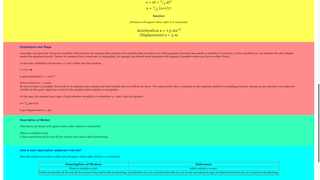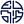Intro Page

1D Kinematics

### Translations

Code Language Translator Run### Software Requirements

SoftwareRequirements

 Android iOS Windows MacOS with best with Chrome Chrome Chrome Chrome support full-screen? Yes. Chrome/Opera No. Firefox/ Samsung Internet Not yet Yes Yes cannot work on some mobile browser that don't understand JavaScript such as..... cannot work on Internet Explorer 9 and below

### CreditsDamian, Robin, Weiqiang

### Sample Learning Goals

An interactive for Kinematics in Physics. Brief Description
• We hope to build a simulator for kinematics in 1D that allows students to input parameters (which can be displacement, initial velocity, final velocity, acceleration, time). With any 3 parameters, the simulator can automatically and quantitatively solve for the other 2 parameters using the kinematic equations.
• Based on the input and solution for all the parameters, the simulator will play out the motion of the object for easy visualization.
• The simulator will also write down the steps (e.g. which equations of motions were used, which terms were substituted etc.) for the students to follow.
• The simulator will also describe the motion in words (e.g. object starts moving to the right and decelerates, it then changes direction and accelerates to the left) so students can learn how to physically describe motion based on the mathematical parameters. This description can be automatically generated in our program based on the parameters (e.g. using IF loops to compare if initial velocity and final velocity are of same sign, or if acceleration is same sign as initial velocity etc.).
Challenges that Students Face in Kinematics
• Students often memorize equations of motion and plug these equations blindly without knowing what they really mean. Sometimes they may get the correct answer by luck.
• Students have difficulty getting the signs correct or even interpreting what the signs mean (e.g. what does negative acceleration means, what happens if both velocity and acceleration are negative etc.).
• Students cannot connect the mathematical parameters describing the motion of objects to the physical motion of an object (especially those with poorer visualization skills). They may solve exam questions and get it correct but may not know how to physically describe what they just solve, in terms of how the object is moving.
Lesson Package that Simulator is Suitable For
• Guided discovery learning can be applied using the simulation for students to explore simulation with instructions.
• Students can be asked to vary parameters in the simulation (e.g. what happens when initial velocity becomes positive, what happens if acceleration becomes larger), that results in the object following different types of motion (e.g. changing direction halfway, accelerating, decelerating).
• They can then fill up a table of observations and how it links to the different parameters (e.g. if initial velocity and final velocity have different signs, it means object changes direction). This allows them to link the mathematical parameters to physical description in a lot of scenarios, which the teacher may not have enough time to cover in class.
• Students can also be purposely asked to fill in parameters that result in no solutions and asked to describe why these parameters have physically impossible outcomes (e.g. if initial velocity is positive, final velocity is negative but acceleration is positive).
• As a challenge, a description of motion for students can even be given to them and students can be asked to come up with the parameters to match this description of motion (students can screenshot the description generated by the simulator to submit their work, or just submit the parameters for the simulator to auto-mark).
Other Uses of Simulator
• Beyond using this simulator for a specially designed lesson package, students can use it any time to check their own understanding by predicting how objects will move based on the parameters they give and then verifying it.
• Weaker students can also try to first look at the motion of the object that the simulator shows after they input the parameters, and then verifying it with the description that the simulator gives, just to improve their description of phenomena in words.
• Some students may also just use it as a quick check if their calculations are correct when solving 1D kinematics problems. If they are wrong, they can immediately refer to the steps automatically generated by the simulator to see what went wrong.
• Some students may also just want to use it to randomly explore further to cover all types of motion.
• Hence simulator is suitable for any stage of learning.
More Ambitious Extension (if compatible with SLS?)
• Using AI, such as those that exist in tensorflow javascript, simulator can automatically compare the description of motion that students type in, with the correct description and auto mark it.
• Even more ambitious, adaptive learning AI to see what student is weak at and give more examples of this kind of motion for the student to analyze.
Rough Plan of How Simulation will Look
Form for students to fill in 3 input parameters such as initial velocity, final velocity, acceleration, time, displacement.
After inputting the parameters, there is a play button or submit button to start the solver.
Animation frame with moving object that shows motion of object after all 5 parameters are solved.
Can have a slider to adjust speed of animation.
Solution to the unknown parameters that simulator has solved.
Steps taken to get to the solution. (e.g. Step 1, use this kinematics equation. Step 2, use the other equation.)
Description of motion of object generated by simulator. (Can hide this first until student click a reveal button)
AI? Another form for student to submit description of motion and how closely it matches the actual one?
Other features.

[text]

### end faq